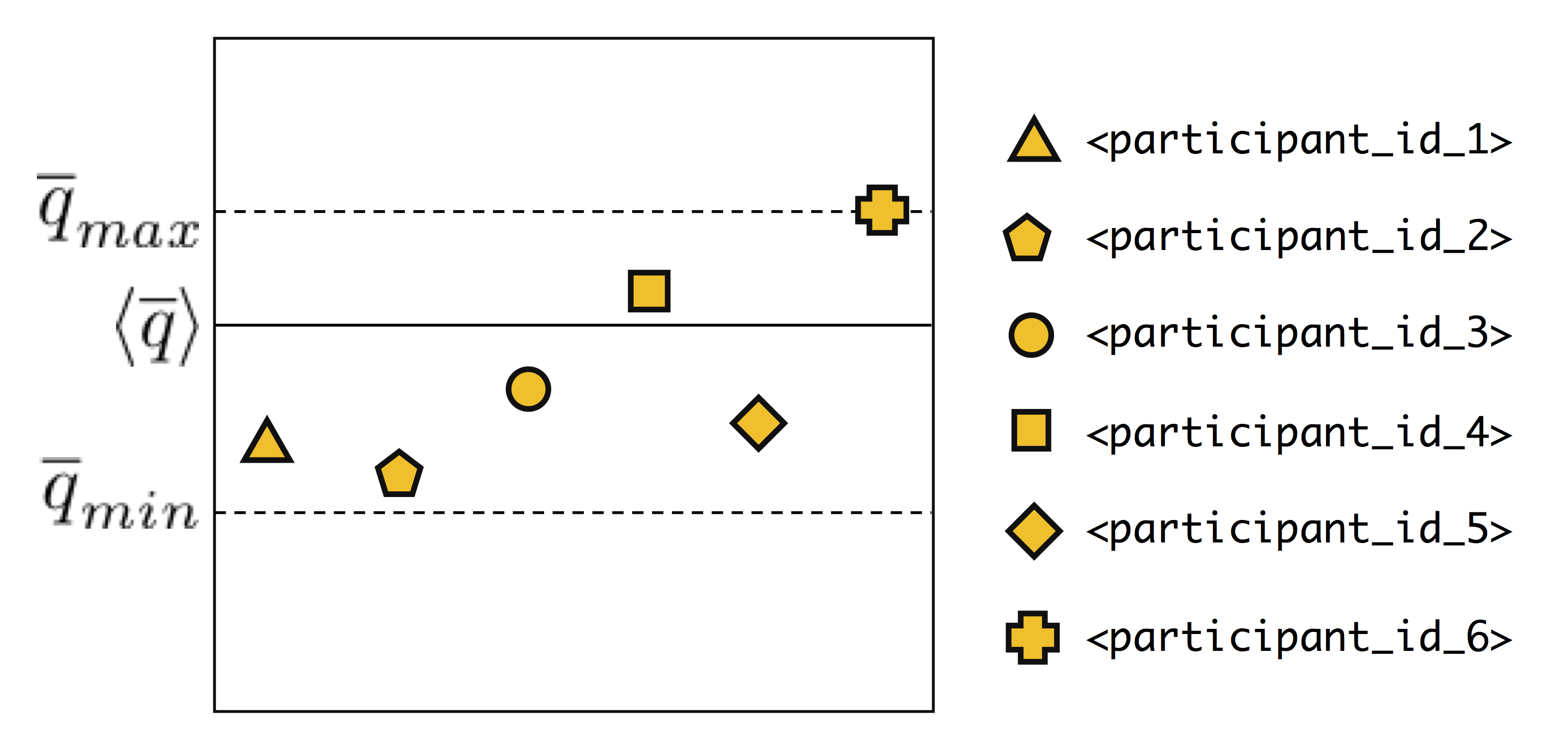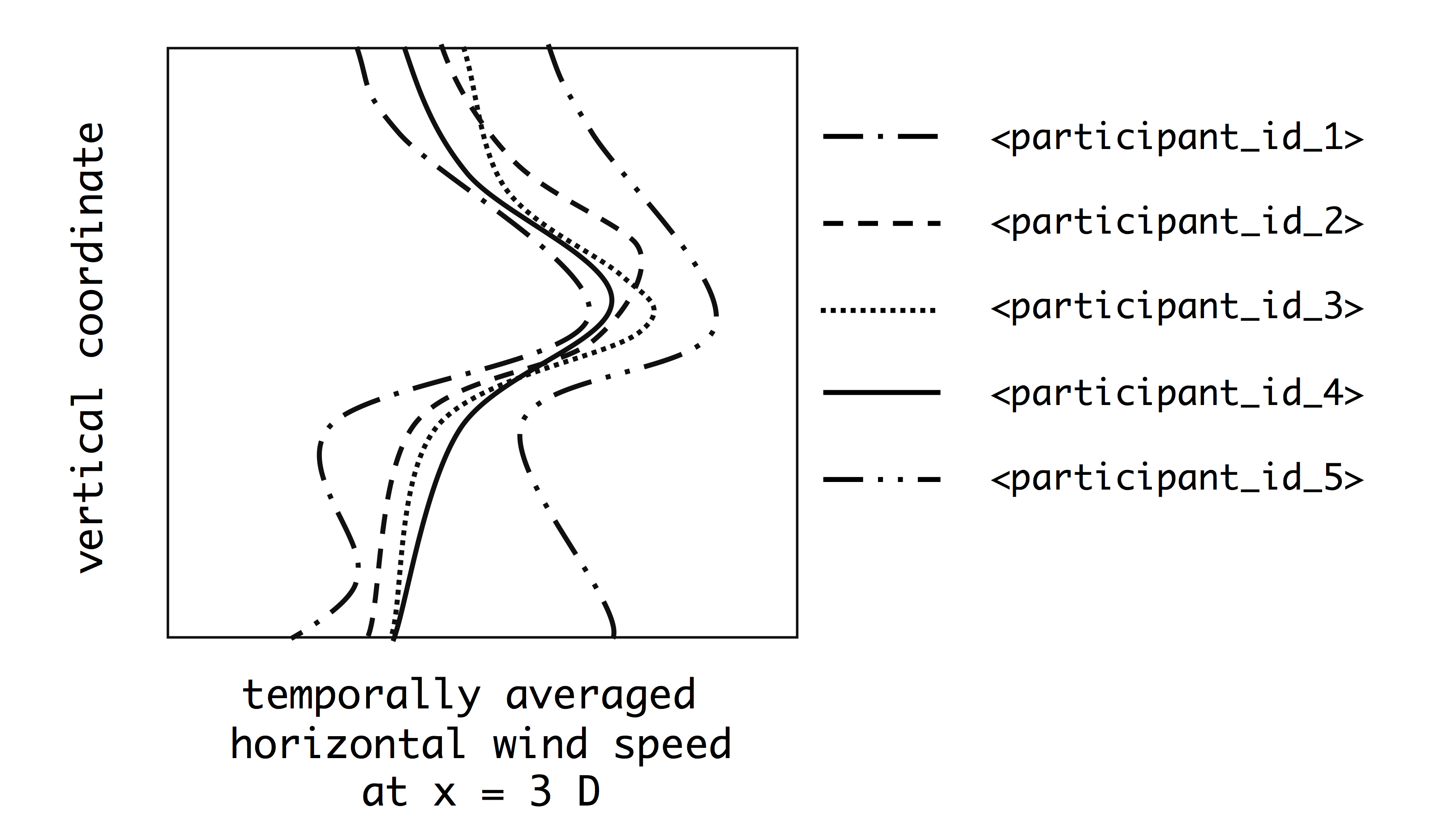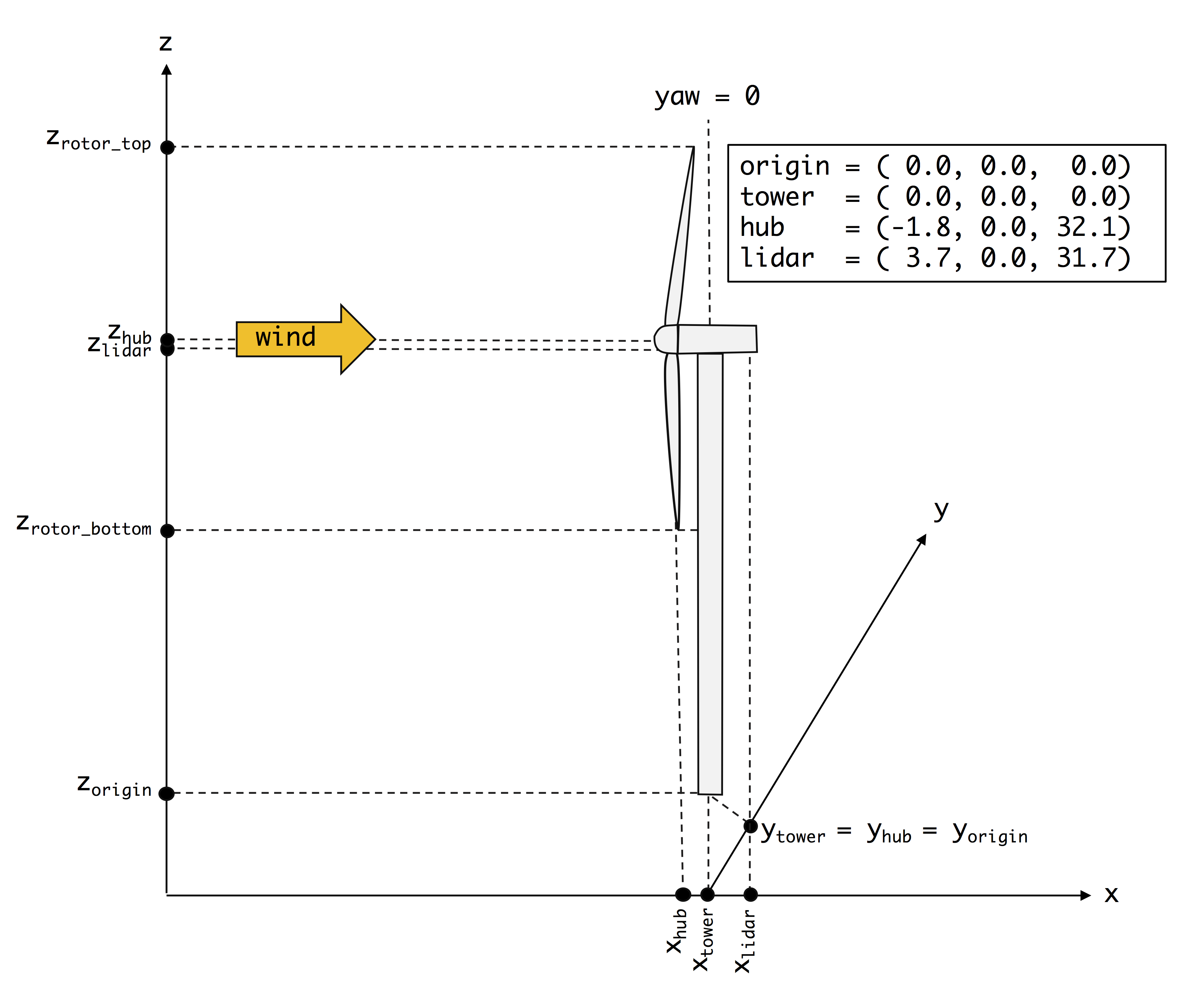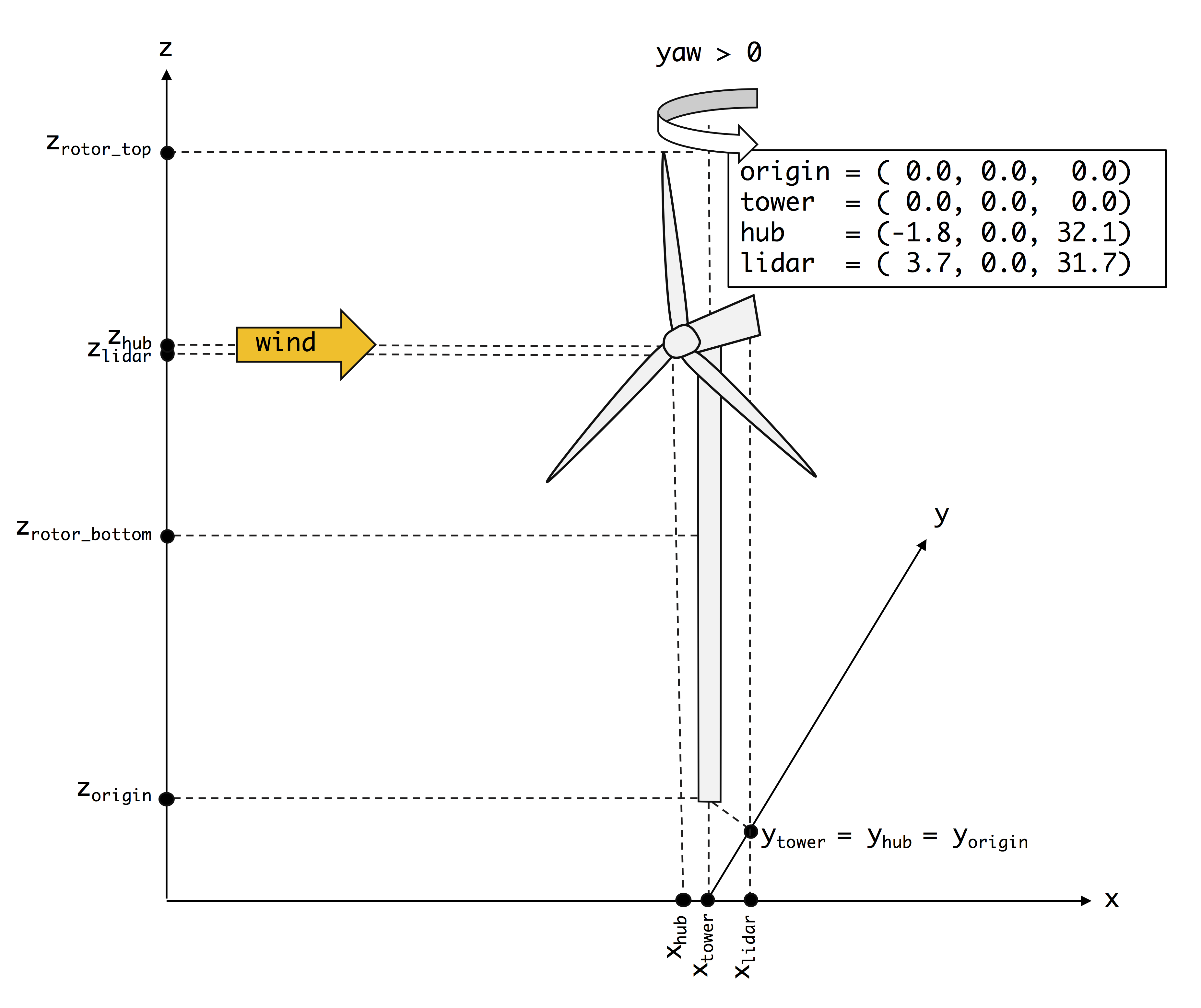# Validation Methodology¶

For variable definitions, refer to the Glossary.

## Atmospheric Inflow Quantities of Interest¶

Simulation results uploaded by the participants will be processed by the benchmark organizers to diagnose atmospheric inflow quantities of interest with the publicly released notebooks (available in this repository).

• $$\overline{U}_{hub}$$
• $$\overline{TI}_{hub}$$
• $$\alpha$$
• $$\overline{\Delta \beta}_{rotor}$$
• $$\sigma_u$$
• $$\sigma_v$$
• $$\sigma_w$$
• $$u_*$$
• $$t_*$$

## Wake Quantities of Interest¶

The uploaded results will be processed by the benchmark organizers to diagnose quantities of interest with the publicly released notebooks and the SAMWICh toolbox. The wake characterization quantities of interest are the following:

• Horizontal profile of temporally averaged horizontal wind speed and velocity deficit at each requested distance downstream
• at $$z=z_{hub}$$ for the fixed frame-of-reference
• at $$z=z_{wake\_center}$$ for the meandering frame-of-reference
• Vertical profile of temporally averaged horizontal wind speed and velocity deficit at each requested distance downstream
• at $$y=y_{hub}$$ for the fixed frame-of-reference
• at $$y=y_{wake\_center}$$ for the meandering frame-of-reference
• Shape of mean wake at each requested distance downstream (qualitative comparison)
• Length scale of mean wake at each requested distance downstream, estimated as the spread parameters (in $$y$$ and $$z$$) of the 2-dimensional Gaussian fit to the velocity-deficit field
• Median value of velocity deficit considering all points within the mean-wake bounds, computed at each requested distance downstream
• Histogram of time series of wake center position in $$y$$ and $$z$$

Note that all of the above quantities will be diagnosed by the benchmark organizers from the time series of flow fields uploaded by the participants.

## Validation Metrics¶

Phase 1: Code Calibration

During the calibration phase, absolute values characterizing atmospheric inflow and turbine operation will be directly compared to the measured values. For each quantity (e.g. temporally averaged hub-height turbulence intensity), the simulation values will be compared to the average, the minimum, and the maximum of the measurement ensemble used to define the benchmark, as illustrated in the schematic.Figure: Schematic of comparison between $$\overline{q}$$ values produced by different codes (markers), the mean of the measurements ensemble $$\langle \overline{q} \rangle$$ (solid line) and the minimum $$\overline{q}_{min}$$ and maximum $$\overline{q}_{max}$$ range of the measurements ensemble (dashed lines)

Phase 2, Part 1: Blind Comparison (Code-to-Code)

Here, the codes are cross-compared without considering the measurements. In this scenario, absolute values of the wake quantities of interest will be compared visually. The objective is to identify gross discrepancies so that simulation results can be improved before the measurement data are released.Figure: Schematic of visual code-to-code comparison for a wake quantity of interest

Phase 2, Part 2: Blind Comparison (Codes-to-Measurements)

Here, the simulation results are compared to the measurements. The visual comparison described above is supplemented by error metrics (e.g., mean error, root-mean-square error).

Phase 3: Iteration

Participants are encouraged to iterate on their results and re-submit them for a comparison to the measurements. In this phase, the visual comparison and error-metric comparison are also performed and the improvement in error metrics is tracked relative to prior submissions of model results by the same participant.

## Coordinate System¶

Unless otherwise noted, all data are analyzed in a coordinate system where $$x$$ is aligned with the mean wind direction as shown in the schematic.Figure: Coordinate system to be used in the validation analyses, when turbine is facing the mean wind. Coordinates (x,y,z) given in meters from origin.Figure: Coordinate system to be used in the validation analyses, when turbine is misaligned with a positive yaw. Coordinates (x,y,z) given in meters from origin.